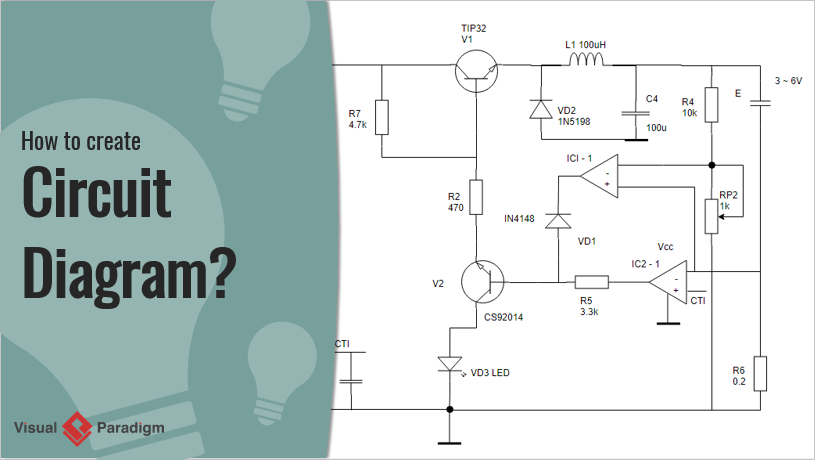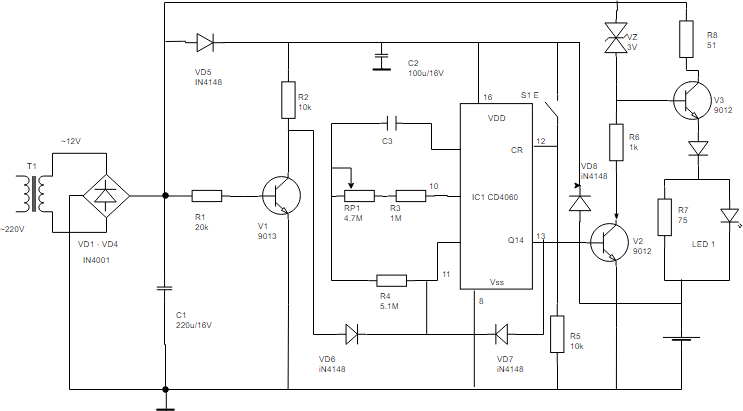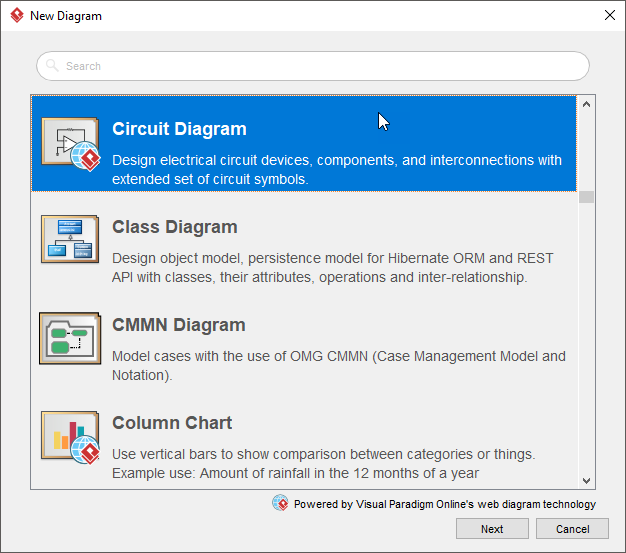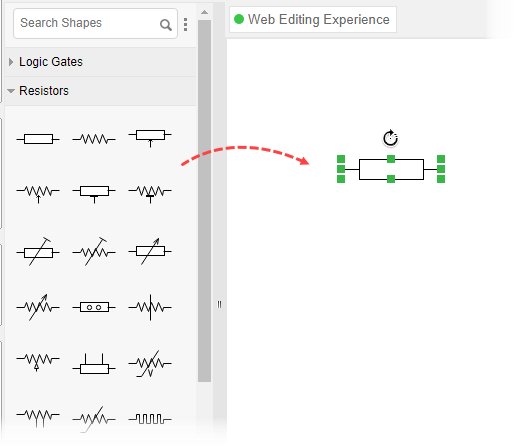# How to Create Circuit Diagram?

Want to create a circuit diagram? We will provide you with an introduction to the circuit diagram and let you know how you can create a circuit diagram with our circuit diagram software.### What is Circuit Diagram?

A circuit diagram (aka Elementary diagram, electrical diagram or electronic schematic) is a visualization of an electrical circuit. It shows the flow and relationships between components in an electrical circuit. Circuit diagrams also visualize the physical arrangement of wires and the components that connect them within different electronic systems.### Purpose of Circuit Diagrams

Circuit diagrams are created as a blueprint of circuit design. Circuit diagram provides a graphical representation of the physical arrangement of all components in a circuit and the wire relationships between them. The technician creates a circuit diagram as a guideline for implementing circuit design or for communication.

### Understanding circuit diagrams

A circuit diagram is a graphical visualization of electrical circuits. It provides a schematic illustration that shows the relationships between components in a circuit. The figure above shows a circuit diagram that provides you with ideas about how circuit diagram symbols look like.

### How to Draw a Circuit Diagram?

1. Select Diagram > New from the main menu.
2. In the New Diagram window, select Circuit Diagram and click Next.3. Select an existing circuit diagram template, or select Blank to create from scratch. Click Next.
4. Enter the diagram name and click OK.
5. Drag and drop the circuit diagram symbol you need from the palette onto the canvas. Connect them with proper connector lines.6. When you finished, you can export the diagram as an image (JPG, PNG, PDF, SVG, etc) and share it with your friends or co-workers (Project > Export > Active Diagram as Image…).